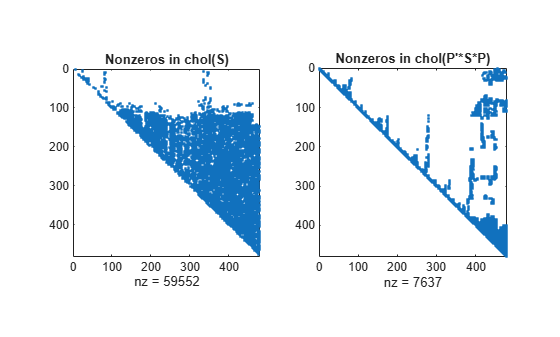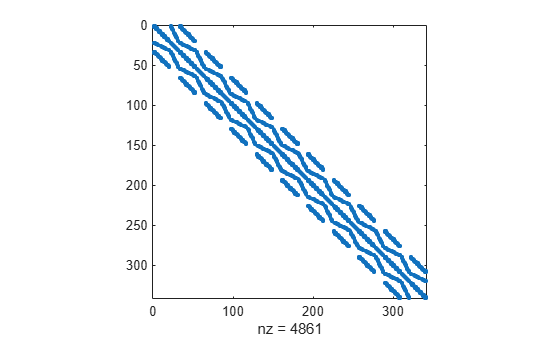# chol

Cholesky factorization

## Syntax

``R = chol(A)``
``R = chol(A,triangle)``
``[R,flag] = chol(___)``
``[R,flag,P] = chol(S)``
``[R,flag,P] = chol(___,outputForm)``

## Description

example

````R = chol(A)` factorizes symmetric positive definite matrix `A` into an upper triangular `R` that satisfies `A = R'*R`. If `A` is nonsymmetric , then `chol` treats the matrix as symmetric and uses only the diagonal and upper triangle of `A`.```

example

````R = chol(A,triangle)` specifies which triangular factor of `A` to use in computing the factorization. For example, if `triangle` is `'lower'`, then `chol` uses only the diagonal and lower triangular portion of `A` to produce a lower triangular matrix `R` that satisfies `A = R*R'`. The default value of `triangle` is `'upper'`.```

example

````[R,flag] = chol(___)` also returns the output `flag` indicating whether `A` is symmetric positive definite. You can use any of the input argument combinations in previous syntaxes. When you specify the `flag` output, `chol` does not generate an error if the input matrix is not symmetric positive definite. If `flag = 0` then the input matrix is symmetric positive definite and the factorization was successful.If `flag` is not zero, then the input matrix is not symmetric positive definite and `flag` is an integer indicating the index of the pivot position where the factorization failed. ```

example

````[R,flag,P] = chol(S)` additionally returns a permutation matrix `P`, which is a preordering of sparse matrix `S` obtained by `amd`. If ```flag = 0```, then `S` is symmetric positive definite and `R` is an upper triangular matrix satisfying ```R'*R = P'*S*P```.```

example

````[R,flag,P] = chol(___,outputForm)` specifies whether to return the permutation information `P` as a matrix or vector, using any of the input argument combinations in previous syntaxes. This option is only available for sparse matrix inputs. For example, if `outputForm` is `'vector'` and `flag = 0`, then ```S(p,p) = R'*R```. The default value of `outputForm` is `'matrix'` such that `R'*R = P'*S*P`.```

## Examples

collapse all

Use `chol` to factorize a symmetric coefficient matrix, and then solve a linear system using the Cholesky factor.

Create a symmetric matrix with positive values on the diagonal.

`A = [1 0 1; 0 2 0; 1 0 3]`
```A = 3×3 1 0 1 0 2 0 1 0 3 ```

Calculate the Cholesky factor of the matrix.

`R = chol(A)`
```R = 3×3 1.0000 0 1.0000 0 1.4142 0 0 0 1.4142 ```

Create a vector for the right-hand side of the equation $\mathrm{Ax}=\mathit{b}$.

`b = sum(A,2);`

Since $\mathit{A}={\mathit{R}}^{\mathit{T}}\mathit{R}$ with the Cholesky decomposition, the linear equation becomes ${\mathit{R}}^{\mathit{T}}\mathit{R}\text{\hspace{0.17em}}\mathit{x}=\mathit{b}$. Solve for `x` using the backslash operator.

`x = R\(R'\b)`
```x = 3×1 1.0000 1.0000 1.0000 ```

Calculate the upper and lower Cholesky factorizations of a matrix and verify the results.

Create a 6-by-6 symmetric positive definite test matrix using the `gallery` function.

`A = gallery('lehmer',6);`

Calculate the Cholesky factor using the upper triangle of `A`.

`R = chol(A)`
```R = 6×6 1.0000 0.5000 0.3333 0.2500 0.2000 0.1667 0 0.8660 0.5774 0.4330 0.3464 0.2887 0 0 0.7454 0.5590 0.4472 0.3727 0 0 0 0.6614 0.5292 0.4410 0 0 0 0 0.6000 0.5000 0 0 0 0 0 0.5528 ```

Verify that the upper triangular factor satisfies `R'*R - A = 0`, within roundoff error.

`norm(R'*R - A)`
```ans = 3.5928e-16 ```

Now, specify the `'lower'` option to calculate the Cholesky factor using the lower triangle of `A`.

`L = chol(A,'lower')`
```L = 6×6 1.0000 0 0 0 0 0 0.5000 0.8660 0 0 0 0 0.3333 0.5774 0.7454 0 0 0 0.2500 0.4330 0.5590 0.6614 0 0 0.2000 0.3464 0.4472 0.5292 0.6000 0 0.1667 0.2887 0.3727 0.4410 0.5000 0.5528 ```

Verify that the lower triangular factor satisfies `L*L' - A = 0`, within roundoff error.

`norm(L*L' - A)`
```ans = 3.6338e-16 ```

Use `chol` with two outputs to suppress errors when the input matrix is not symmetric positive definite.

Create a 5-by-5 matrix of binomial coefficients. This matrix is symmetric positive definite, so subtract 1 from the last element to ensure it is no longer positive definite.

```A = pascal(5); A(end) = A(end) - 1```
```A = 5×5 1 1 1 1 1 1 2 3 4 5 1 3 6 10 15 1 4 10 20 35 1 5 15 35 69 ```

Calculate the Cholesky factor for `A`. Specify two outputs to avoid generating an error if `A` is not symmetric positive definite.

`[R,flag] = chol(A)`
```R = 4×4 1 1 1 1 0 1 2 3 0 0 1 3 0 0 0 1 ```
```flag = 5 ```

Since `flag` is nonzero, it gives the pivot index where the factorization fails. `chol` is able to calculate `q = flag-1 = 4` rows and columns correctly before failing when it encounters the part of the matrix that changed.

Verify that `R'*R` returns four rows and columns that agree with `A(1:q,1:q)`.

```q = flag-1; R'*R```
```ans = 4×4 1 1 1 1 1 2 3 4 1 3 6 10 1 4 10 20 ```
`A(1:q,1:q)`
```ans = 4×4 1 1 1 1 1 2 3 4 1 3 6 10 1 4 10 20 ```

Calculate the Cholesky factor of a sparse matrix, and use the permutation output to create a Cholesky factor with fewer nonzeros.

Create a sparse positive definite matrix based on the `west0479` matrix.

```load west0479 A = west0479; S = A'*A;```

Calculate the Cholesky factor of the matrix two different ways. First specify two outputs, and then specify three outputs to enable row and column reordering.

```[R,flag] = chol(S); [RP,flagP,P] = chol(S);```

For each calculation, check that `flag = 0` to confirm the calculation is successful.

```if ~flag && ~flagP disp('Factorizations successful.') else disp('Factorizations failed.') end```
```Factorizations successful. ```

Compare the number of nonzeros in `chol(S)` vs. the reordered matrix `chol(P'*S*P)`. Best practice is to use the three output syntax of `chol` with sparse matrices, since reordering the rows and columns can greatly reduce the number of nonzeros in the Cholesky factor.

```subplot(1,2,1) spy(R) title('Nonzeros in chol(S)') subplot(1,2,2) spy(RP) title('Nonzeros in chol(P''*S*P)')```Use the `'vector'` option of `chol` to return the permutation information as a vector rather than a matrix.

Create a sparse finite element matrix.

```S = gallery('wathen',10,10); spy(S)```Calculate the Cholesky factor for the matrix, and specify the `'vector'` option to return a permutation vector `p`.

`[R,flag,p] = chol(S,'vector');`

Verify that `flag = 0`, indicating the calculation is successful.

```if ~flag disp('Factorization successful.') else disp('Factorization failed.') end```
```Factorization successful. ```

Verify that `S(p,p) = R'*R`, within roundoff error.

`norm(S(p,p) - R'*R,'fro')`
```ans = 2.1039e-13 ```

## Input Arguments

collapse all

Input matrix. Argument `A` can use full or sparse storage, but must be square and symmetric positive definite.

`chol` assumes that `A` is symmetric for real matrices or Hermitian for complex matrices. `chol` uses only the upper or lower triangle of `A` to perform its computations, depending on the value of `triangle`.

Data Types: `single` | `double`
Complex Number Support: Yes

Sparse input matrix. `S` must be square and symmetric positive definite.

`chol` assumes that `S` is symmetric for real matrices or Hermitian for complex matrices. `chol` uses only the upper or lower triangle of `S` to perform its computations, depending on the value of `triangle`.

Data Types: `double`
Complex Number Support: Yes

Triangular factor of input matrix, specified as `'upper'` or `'lower'`. Use this option to specify that `chol` should use the upper or lower triangle of the input matrix to compute the factorization. `chol` assumes that the input matrix is symmetric for real matrices or Hermitian for complex matrices. `chol` uses only the upper or lower triangle to perform its computations.

Using the `'lower'` option is equivalent to calling `chol` with the `'upper'` option and the transpose of the input matrix, and then transposing the output `R`.

Example: `R = chol(A,'lower')`

Shape of permutation output, specified as `'matrix'` or `'vector'`. This flag controls whether the permutation output `P` is returned as a permutation matrix or permutation vector.

• If `flag = 0`, then `S` is symmetric positive definite and `P'*S*P = R'*R` (if `P` is a matrix) or `S(p,p) = R'*R` (if `p` is a vector).

• If `flag` is not zero, then `S` is not symmetric positive definite. `R` is an upper triangular matrix of size `q`-by-`n`, where ```q = flag-1```. The L-shaped region of the first `q` rows and first `q` columns of `R'*R` agree with those of `P'*S*P` (if `P` is a matrix) or `S(p,p)` (if `p` is a vector).

• If the `'lower'` option is specified, then `R` is a lower triangular matrix and you can replace `R'*R` with `R*R'` in the previous identities.

The Cholesky factor of `P'*S*P` (if `P` is a matrix) or `S(p,p)` (if `p` is a vector) tends to be sparser than the Cholesky factor of `S`.

Example: `[R,flag,p] = chol(S,'vector')`

## Output Arguments

collapse all

Cholesky factor, returned as a matrix.

• If `R` is upper triangular, then `A = R'*R`. If you specify the `P` output for sparse matrices, then `P'*S*P = R'*R` or `S(p,p) = R'*R`, depending on the value of `outputForm`.

• If `R` is lower triangular, then `A = R*R'`. If you specify the `P` output for sparse matrices, then `P'*S*P = R*R'` or `S(p,p) = R*R'`, depending on the value of `outputForm`..

• Whenever `flag` is not zero, `R` contains only partial results. `flag` indicates the pivot position where the factorization failed, and `R` contains the partially completed factorization.

Symmetric positive definite flag, returned as a scalar.

• If `flag = 0`, then the input matrix is symmetric positive definite. `R` is an upper triangular matrix such that ```R'*R = A```.

• If `A` is not symmetric positive definite, then `flag` is a positive integer indicating the pivot position where the factorization failed, and MATLAB® does not generate an error. `R` is an upper triangular matrix of size `q = flag-1` such that ```R'*R = A(1:q,1:q)```.

• If `A` is sparse, then `R` is an upper triangular matrix of size `q`-by-`n` such that the L-shaped region of the first `q` rows and first `q` columns of `R'*R` agree with those of `A` or `S`.

• If the `'lower'` option is specified, then `R` is a lower triangular matrix and you can replace `R'*R` with `R*R'` in the previous identities.

Permutation for sparse matrices, returned as a matrix or vector depending on the value of `outputForm`. See `outputForm` for a description of the identities that this output satisfies.

This permutation matrix is based on the approximate minimum degree ordering computed by `amd`. However, this preordering can differ from the one obtained directly by `amd` since `chol` slightly changes the ordering for increased performance.

collapse all

### Symmetric Positive Definite Matrix

A symmetric positive definite matrix is a symmetric matrix with all positive eigenvalues.

For any real invertible matrix `A`, you can construct a symmetric positive definite matrix with the product `B = A'*A`. The Cholesky factorization reverses this formula by saying that any symmetric positive definite matrix `B` can be factored into the product `R'*R`.

A symmetric positive semi-definite matrix is defined in a similar manner, except that the eigenvalues must all be positive or zero.

The line between positive definite and positive semi-definite matrices is blurred in the context of numeric computation. It is rare for eigenvalues to be exactly equal to zero, but they can be numerically zero (on the order of machine precision). For this reason, `chol` might be able to factorize one positive semi-definite matrix, but could fail with another matrix that has very similar eigenvalues.

## Version History

Introduced before R2006a